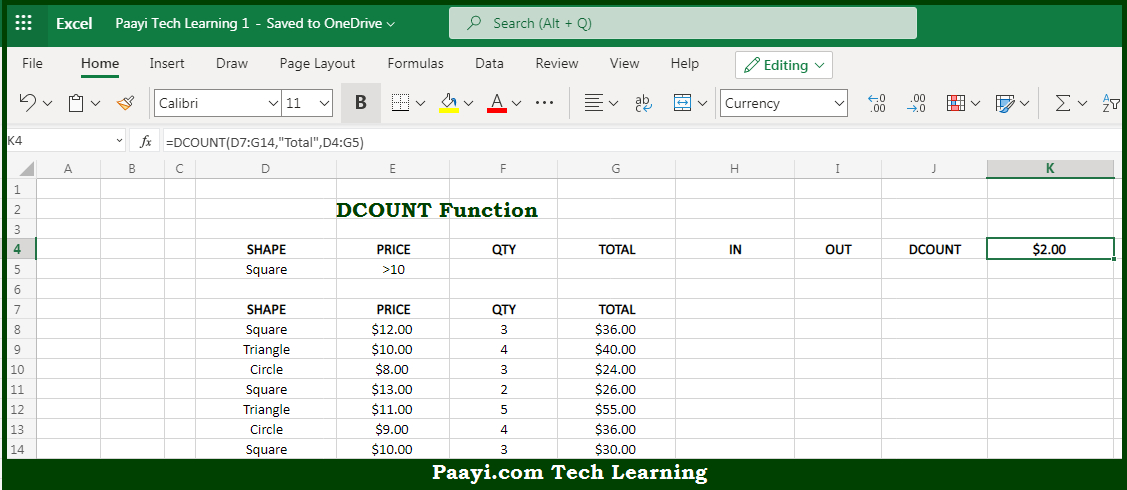# Learn How to Use Microsoft Excel DCOUNT Function

Written by | 0 Comments | 467 Views

In this article, you will learn how to use the Microsoft Excel DCOUNT function and its prime function in Microsoft Excel. You will also get to know the Microsoft Excel DCOUNT function return value and syntax with the help of some examples.

Microsoft Excel DCOUNT Function

The main purpose of the Microsoft Excel DCOUNT function is to count the matching records in the database. That implies, with the help of DCOUNT function you can able to count matching records in a database using criteria and an optional field. It should be noted that when a field is provided the DCOUNT function will only count numeric values in the field. You can use DCOUNTA function to count numbers or text values in a given field. So, with the help of DCOUNT function, you can able to count the matching records in the database.

Return Value of DCOUNT Function

The return value will be the numeric count of matching records.

Syntax of DCOUNT Function

=DCOUNT(database, [field], criteria)

Where the arguments:

• database: This is the database range including headers.
• field: This is the field name or index to count (optional).
• criteria: This is the criteria range including headers.

## How to Use Microsoft Excel DCOUNT Function?So we know that Microsoft Excel DCOUNT function you can able to count the matching records in the database. That implies, with the help of DCOUNT function you can able to count matching records in a database using criteria and an optional field. It should be noted that when a field is provided the DCOUNT function will only count numeric values in the field. You can use DCOUNTA function to count numbers or text values in a given field. So, with the help of DCOUNT function, you can able to count the matching records in the database.